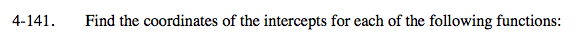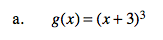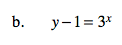### Home > AC > Chapter 13 > Lesson 13.CS1-S > Problem4-141

4-141.y-intercept where x = 0:

g(0) = (0 + 3)3

g(0) = 27

x-intercept where g(x) = 0:

0 = (x + 3)3

$\sqrt{0}=(\textit{x}+3)$

−3 = x

The y-intercept is at (0, 27).
The x-intercept is at (−3, 0).See part (a).